Hello Guest it is May 15, 2021, 08:27:51 PM

###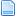Author Topic: Feed Rate Override Absolute Value?  (Read 2941 times)

0 Members and 1 Guest are viewing this topic.

####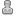Cal892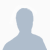•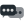12##### Re: Feed Rate Override Absolute Value?
« Reply #10 on: December 22, 2016, 10:13:01 AM »
I have three DRO's; one for X move value, one for Y move, and one for feed rate. The feed rate entered is an absolute feed rate so the feed rate is determined as a percentage of 150in/min(100% jog speed on my setup)
After calculating the diagonal distance needed to travel, feed rate values are calculated for the X and Y so that the motors move together and arrive at the same point at the same time. the values are factors of the Hypotenuse so as to create the right triangle.

For some reason I was originally having issues with setting slow feed rates because the motors were running at rapid feed rates and the PMDX BOB was sending strange signals resulting in the motors moving abnormally slowly(up to 10x slower than was entered). After the jog rate was corrected in the configurations it ran smoothly.

The script is all in the "left up" section, there are probably more tonumber() functions than are necessary, but I was having some problems and haven't gone back to remove extras as they don't cause any issues.

Code: [Select]
`--"Move To" Scriptlocal inst = mc.mcGetInstance()local hypLength = 0                                 local rateH = scr.GetProperty("droSpeed", "Value")  --Requires DRO named "droSpeed"local rateX = 0local rateY = 0local xJog = scr.GetProperty("droXmove", "Value")   --Requires DRO named "droXmove"local yJog = scr.GetProperty("droYmove", "Value")   --Requires DRO named "droYmove"local xNeg = 1local yNeg = 1hypLength = tonumber(hypLength)rateH = tonumber(rateH)rateX = tonumber(rateX)rateY = tonumber(rateY)xJog = tonumber(xJog)yJog = tonumber(yJog)xNeg = tonumber(xNeg)yNeg = tonumber(yNeg)--Determining if negative valuesif(xJog < 0) then xNeg = -1math.abs(xJog)endif(yJog < 0) thenyNeg = -1math.abs(yJog)end--X Y Rates CalchypLength = math.sqrt((xJog^2)+(yJog^2))rateX = xJog/hypLengthrateX = rateH*rateXrateY = yJog/hypLengthrateY = rateH*rateY--Feed rate calcs if single asix travel(100% = 150in/min)if(xJog or yJog == 0) thenrateH = rateH/1.50rateH = tonumber(rateH)rateX = rateHrateY = rateHend--Set jog rate for each axis rateX = tonumber(rateX)rateY = tonumber(rateY)mc.mcJogSetRate(inst, 0, rateX)mc.mcJogSetRate(inst, 1, rateY)--Input distance to travel for each axisxJog = tonumber(xJog)yJog = tonumber(yJog)xJog = xJog*xNegyJog = yJog*yNegmc.mcJogIncStart(inst, 0, xJog)mc.mcJogIncStart(inst, 1, yJog)`
Thanks again for all the help, I hope this can be of use to others as well

####Chaoticone•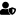•5,626
• Precision Chaos##### Re: Feed Rate Override Absolute Value?
« Reply #11 on: December 22, 2016, 02:41:24 PM »
Nice! I'lll check out your script later today. Thanks for posting your solution.If you could see the things I have in my head, you would be laughing too.My guard dog is not what you need to worry about!

####Cal892•12##### Re: Feed Rate Override Absolute Value?
« Reply #12 on: January 06, 2017, 04:56:37 PM »
I realized there were some errors in the code posted earlier. Here is an update.

Code: [Select]
`--"Move To" Scriptlocal inst = mc.mcGetInstance()local hypLength = 0                                 local rateH = scr.GetProperty("droSpeed", "Value")  --Requires DRO named "droSpeed"local rateX = 0local rateY = 0local xJog = scr.GetProperty("droXmove", "Value")   --Requires DRO named "droXmove"local yJog = scr.GetProperty("droYmove", "Value")   --Requires DRO named "droYmove"local xNeg = xJoglocal yNeg = yJoghypLength = tonumber(hypLength)rateH = tonumber(rateH)rateX = tonumber(rateX)rateY = tonumber(rateY)xJog = tonumber(xJog)yJog = tonumber(yJog)xNeg = tonumber(xNeg)yNeg = tonumber(yNeg)rateH = rateH/1.50--Determining if negative valuesif(xJog < 0) then --xNeg = xJogmath.abs(xJog)endif(yJog < 0) then--yNeg = yJogmath.abs(yJog)end--X Y Rates CalchypLength = math.sqrt((xJog^2)+(yJog^2))rateX = xJog/hypLengthrateX = rateH*rateXrateY = yJog/hypLengthrateY = rateH*rateY--Set jog rate for each axis rateX = tonumber(rateX)rateY = tonumber(rateY)mc.mcJogSetRate(inst, 0, rateX)mc.mcJogSetRate(inst, 1, rateY)--Input distance to travel for each axisxJog = xNegyJog = yNegmc.mcJogIncStart(inst, 0, xJog)mc.mcJogIncStart(inst, 1, yJog)`